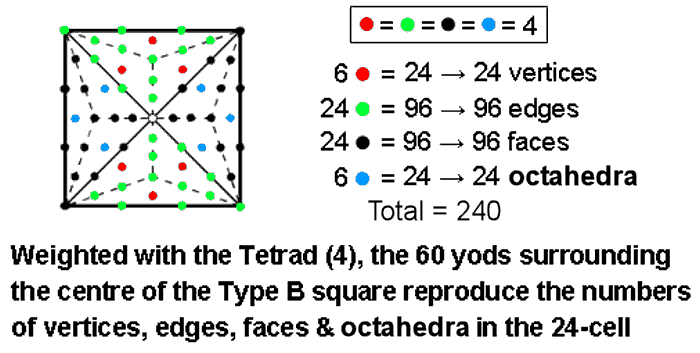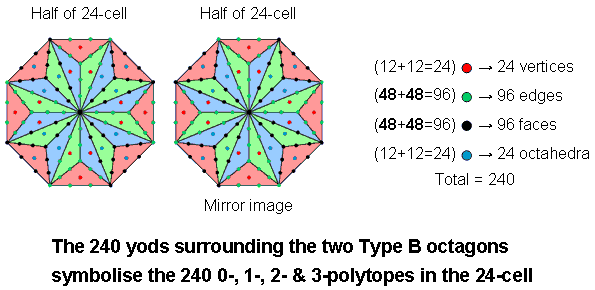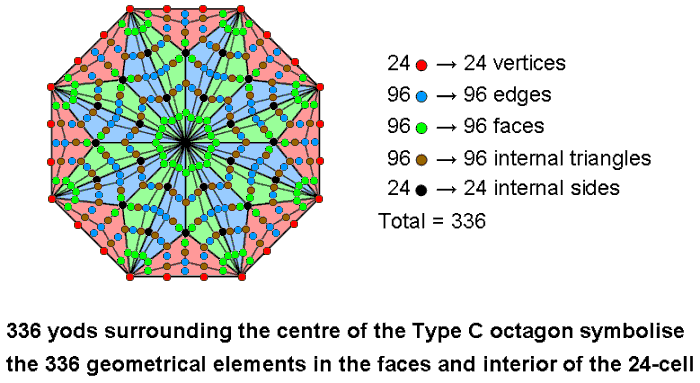Home

 << Previous    1...   20  21    23  24    Next >>

22. How the Type B square and octagon embody the numbers of 0-, 1-, 2- & 3-polytopes in the 24-cellThe square is the symbol of the Tetrad (4). Weighted with the Tetrad, the 60 yods surrounding the centre of the Type B square generate the number 240. This is the number of vertices, (0-polytopes), edges (1-polytopes), faces (2-polytopes) & octahedra (3-polytopes) in the 24-cell. However, this square embodies not only the 240 polytopes making up the 24-cell but also the numbers of each type. As each yod denotes four similar polytopes, the 12 vertices in one half of the 24-cell and the 12 vertices in its other half are denoted by three red yods in one sector and their mirror images in the inverted sector. Similarly, the 12 octahedra in one half and the 12 octahedra in the opposite half of the 24-cell are denoted by three blue yods in one sector and their mirror images in its inverted counterpart. The 48 edges in each half are denoted by 12 green yods in one sector and by 12 green yods in its inverted counterpart. The 48 faces in each half are denoted by 12 black yods in one sector and by 12 black yods in its inverted counterpart.

As 4 = 2×2, the weight of 4 can be removed from each yod by considering the 240 yods that surround the centres of two separate Type B octagons. Each yod now denotes a distinct 0-, 1-, 2- & 3-polytope. One octagon with 120 yods surrounding its centre represents half of the 24-cell with 120 polytopes (12 vertices, 48 edges, 48 faces & 12 octahedra); the second octagon with 120 yods surrounding its centre represents the other half of the 24-cell. It is depicted above by the mirror image of the first octagon.

The Type C octagon has 336 yods surrounding its centre. They symbolise the 336 geometrical elements in the faces and interior of the 24-cell that surround its centre when the latter is joined to its vertices by straight lines:24-cellThe Type A octagon has 24 red yods on its boundary and 24 black yods in its interior surrounding its centre. They denote the 24 vertices and 24 sides of the 96 internal triangles formed by joining vertices to its centre. In the Type C octagon, 36 more yods are added in each sector, 12 blue yods denoting faces, 12 green yods denoting faces and 12 brown yods denoting internal triangles.

It is remarkable that the Type A octagon represents the vertices and sides of internal triangles of the 24-cell and that the Type B octagon represents its polytopic composition, whilst the Type C octagon represents its geometrical composition in terms of points, lines & triangles. A sceptic's suggestion that both properties of the octagon could arise by chance is improbable in the extreme. Moreover, it ignores the fact, confirmed in this website by many analyses of sacred geometries, that both the number 240 (number of polytopes) and the number 336 (number of geometrical elements) characterising the 24-cell are parameters of holistic systems. For examples, see The holistic pattern. It should therefore not be at all surprising that these holistic parameters manifest in the 24-cell, which this section has demonstrated is a holistic system with properties exhibiting the universal patterns found in sacred geometries. Nor should it be unexpected that various regular polygons embody these particular numbers, for they embody other holistic parameters, as demonstrated in many pages of this website.

Anyone who has come to this page without reading the analysis of the 421 polytope and the 24-cell in previous pages may ask: why should the Tetrad and its square symbol pick out the 24-cell? What is the significance of this particular polytope? As pointed out on page 3, the 600-cell is a compound of five 24-cells that comprise (5×336=1680) geometrical elements surrounding their common centre. The 4-dimensional projection of the 421 polytope is a compound of two 600-cells, i.e., 10 24-cells made up of (2×1690=3360) geometrical elements. This is the geometrical counterpart of the fact that every half-revolution of all 10 whorls of the UPA comprises 1680 circular turns, each turn being a circularly polarised oscillation, i.e., a superposition of two orthogonal plane waves that differ in phase by 90°, so that each half-revolution comprises 3360 cycles of two superposed, sinusoidal plane waves. The 24-cell is fundamental to the space-time description of the UPA — hence its representation by the square as the symbol of the Tetrad.

 << Previous    1...   20  21    23  24    Next >>

Home